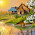In practice, the electric field intensity is not uniform but varies from point to point in an electric field. Let the electric field strength at any point A in an electric field be E (N-C). Now, the unit positive charge at point A is displaced by distance by dx metres in the direction of the field so that the electric field strength remains constant. Work done in moving this charge can be determined by force x displacement.
Work done = + E x dx   joules
Let dV be the potential drop over this distance in the direction of the electric field. It is moved from point of higher potential to lower potential. So, by the definition of a potential difference,

The term dV/dx  in the above expression is called the potential gradient.
Note: potential gradient is defined as the drop in potential per metre in the direction of the electric field.
It is measured in units volts/metre (V/m).
If the change in potential is from lower potential to higher potential, i.e. against the direction of the electric field then potential is said to be negative i.e.

Note: from the above expression, it follows that numerically,
Electric field strength = Potential gradient

#### 1 comment:

1.hi dear its blog very nice and helpful thanks from inverter